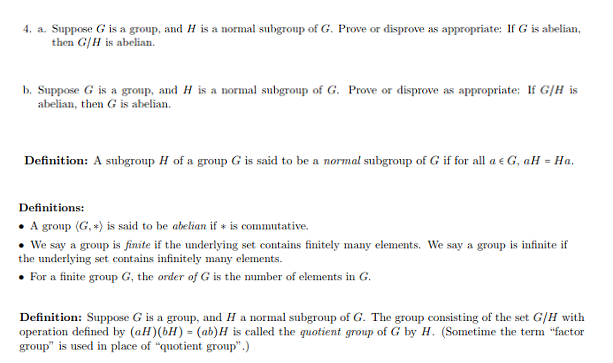# 4. a. Suppose G is a group, and H is a normal subgroup of G. Prove or disprove as appropriate: If G is abelianthen G/H is abelianb. Suppose G is a group, and H is a normal subgroup of G. Prove or disprove as appropriate: I G/H isabelian, then G is abelianDefinition: A subgroup H of a group G is said to be a normal subgroup of G if for all ae G, aH-HaDefinitions:. A group (G,) is said to be abelian ifis commutative.We say a group is finite if the underlying set contains finitely many elements. We say a group is infinite ifthe underlying set contains infinitely many elementsFor a finite group G, the order of G is the number of elements in GDefinition: Suppose G is a group, and H a normal subgroup of G. The group consisting of the set G/H withoperation defined by (aH) (bH) (ab)H is called the quotient group of G by H. (Sometime the term "factorgroup" is used in place of "quotient group)

Question
45 views

Abstract Algebra Proofs:

Looking for help.help_outlineImage Transcriptionclose4. a. Suppose G is a group, and H is a normal subgroup of G. Prove or disprove as appropriate: If G is abelian then G/H is abelian b. Suppose G is a group, and H is a normal subgroup of G. Prove or disprove as appropriate: I G/H is abelian, then G is abelian Definition: A subgroup H of a group G is said to be a normal subgroup of G if for all ae G, aH-Ha Definitions: . A group (G,) is said to be abelian if is commutative. We say a group is finite if the underlying set contains finitely many elements. We say a group is infinite if the underlying set contains infinitely many elements For a finite group G, the order of G is the number of elements in G Definition: Suppose G is a group, and H a normal subgroup of G. The group consisting of the set G/H with operation defined by (aH) (bH) (ab)H is called the quotient group of G by H. (Sometime the term "factor group" is used in place of "quotient group) fullscreen
check_circle

Step 1

To prove or disprove (by a counter example) the given statements on quotient groups

Step 2

4a) We will prove that if G is abelian, then G/H is also abelian. To start with , we show that any subgroup H of an abelian group is normal, so the quotient group G/H always makes sense,

Step 3

a) Next , we show that if G is abelian, H a sub...

### Want to see the full answer?

See Solution

#### Want to see this answer and more?

Solutions are written by subject experts who are available 24/7. Questions are typically answered within 1 hour.*

See Solution
*Response times may vary by subject and question.
Tagged in

### Math